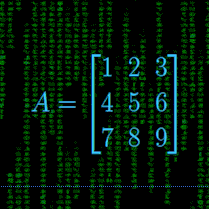﻿ Linear Algebra

# Linear Algebra Methods and Software

## "computer techniques and methods"

This section contains links to a variety of linear algebra methods and solvers. Some of the software is ported to C or C++ from other languages and some is unique or unavailable elsewhere.

The contents of some of the navigation subsections above are:

• NON-LINEAR — non-linear solvers, including Newton's and Broyden's,
• INTEGRATION — integrators, including QUADPACK and double exponential,
• ROOTS — advanced polynomial root-finders, original methods and ports,
• XBCD-MATH — an extended precision BCD math package for calculator use,
• PARSERS — a collection of math expression parsers: double and complex.## C Ports from Linpack

• DGESL -- solves A * X = B or TRANS(A) * X = B using the factors computed by DGECO or DGEFA.
• DGEFA -- factors a real matrix by Gaussian elimination.
• DGEDI -- computes the determinant and inverse of a matrix using the factors computed by DGECO or DGEFA.
• DGTSL -- given a general tridiagonal matrix and a right hand side will find the solution.
• DGECO -- factors a real matrix by Gaussian elimination and estimates the condition of the matrix.
• DPOFA -- factors a real symmetric positive definite matrix.
• DPOSL -- solves the real symmetric positive definite matrix A * X = B using the factors computed by DPOFA.

There is also a header file available. These C programs are functional equivalents to some of the double precision FORTRAN versions. The intent was to port the original code rather than rewrite it, so there are weaknesses including extensive use of the now-disparaged 'goto's. The manual is copyrighted, so you'll have to find it using an internet search. Then grab your trusty Linpack manual for documentation and you're on your way. Note that Linpack relies on the BLAS for low level vector operations. In this port, the equivalent code is inserted directly into each routine.

## C Ports from Handbook for Automatic Computation

These are ports from Algol to C. The differences in the languages are such that it is much easier to port from Algol to C than from FORTRAN to C. As above, the documentation is copyrighted and use of these ports requires the 'Handbook'.

## Original C++ Code for Linear Systems

• GED -- Gaussian elimination routine
• CGED -- complex version of Gaussian elimination routine
• MATINV -- bare-bones (simple pivoting) matrix inverse using Gauss-Jordan elimination
• CMATINV -- complex version of matrix inverse using Gauss-Jordan elimination

## Assembler Language (Tasm32) Linear Solvers

Here is a set of Gaussian elimination routines for float, double and long double numeric types. A header file for these routines is here.